# 27 Results

View
Selected filters:
• Integers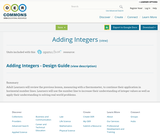Conditions of Use:
No Strings Attached
Rating

Adult Learners will review the previous lesson, measuring with a thermometer, to continue their application in horizontal number lines. Learners will use the number line to increase their understanding of integer values as well as apply their understanding to solving real world problems.

Subject:
Education
Mathematics
Material Type:
Interactive
Lesson Plan
Student Guide
Author:
Lisa Ramsey
05/14/2016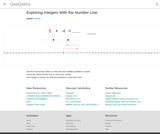Conditions of Use:
Remix and Share
Rating

This simulation allows you to use a slider to see how positive and negative integers add on a number line.

Subject:
Mathematics
Algebra
Numbers and Operations
Material Type:
Diagram/Illustration
Simulation
Provider:
GeoGebra
Author:
rsnyder
05/10/2018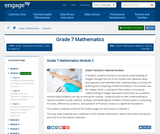Conditions of Use:
Remix and Share
Rating

In Grade 6, students formed a conceptual understanding of integers through the use of the number line, absolute value, and opposites and extended their understanding to include the ordering and comparing of rational numbers. This module uses the Integer Game: a card game that creates a conceptual understanding of integer operations and serves as a powerful mental model students can rely on during the module.  Students build on their understanding of rational numbers to add, subtract, multiply, and divide signed numbers. Previous work in computing the sums, differences, products, and quotients of fractions serves as a significant foundation.

Subject:
Numbers and Operations
Material Type:
Module
Provider:
New York State Education Department
Provider Set:
EngageNY
09/25/2013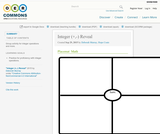Conditions of Use:
Remix and Share
Rating

Group activity for integer operations and more.

Subject:
Mathematics
Material Type:
Activity/Lab
Author:
Deborah Murray
Hope Coats
01/28/2016
Remix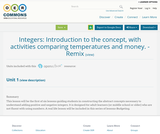Conditions of Use:
No Strings Attached
Rating

This lesson will be the first of six lessons guiding students in constructing the abstract concepts necessary to understand adding positive and negative integers.
It is designed for adult learners (or middle school or older) who are not fluent with using numbers. A real life lesson will be included in this series of lessons-Budgeting.

Subject:
Mathematics
Material Type:
Lesson Plan
Author:
Lynne Alexander
08/08/2019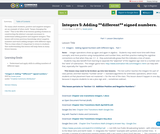Conditions of Use:
No Strings Attached
Rating

For many adult students, positive and negative integers are an example of when math “keeps changing the rules.” This is the fifth of six lessons guiding students in constructing the abstract concepts necessary to understand adding positive and negative integers.
This lesson will review previous knowledge about negative numbers and teach adding integers with different signs. This is a time when it’s important to stress to students that understanding this lesson will help them in many future lessons.

Subject:
Mathematics
Material Type:
Lesson Plan
Author:
Susan Jones
11/03/2017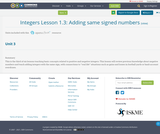Conditions of Use:
No Strings Attached
Rating

This is the third of six lessons teaching basic concepts related to positive and negative integers. This lesson will review previous knowledge about negative numbers and teach adding integers with the same sign, with connections to “real life” situations such as gains and losses in football yards or bank account overdraws.

Subject:
Mathematics
Material Type:
Activity/Lab
Lecture Notes
Teaching/Learning Strategy
Author:
Susan Jones
08/28/2017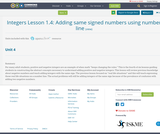Conditions of Use:
No Strings Attached
Rating

For many adult students, positive and negative integers are an example of when math “keeps changing the rules.” This is the fourth of six lessons guiding students in constructing the abstract concepts necessary to understand adding positive and negative integers.
This lesson will review previous knowledge about negative numbers and teach adding integers with the same sign. The previous lesson focused on “real life situations” and this will teach expressing those real life situations on a number line. The actual problems will still be adding integers of the same sign because of the prevalence of confusion with adding two negative numbers.

Subject:
Mathematics
Material Type:
Activity/Lab
Lecture Notes
Teaching/Learning Strategy
Author:
Susan Jones
09/13/2017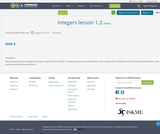Conditions of Use:
No Strings Attached
Rating

This second lesson in negative integers carries the "real life" concept (temperature and debt) to the number line. Students will work with number lines with positive and negative numbers.

Subject:
Mathematics
Material Type:
Activity/Lab
Homework/Assignment
Author:
Susan Jones
03/10/2018Conditions of Use:
Remix and Share
Rating

Subject:
Mathematics
Material Type:
Full Course
Provider:
Pearson
10/06/2016Conditions of Use:
Remix and Share
Rating

Rational Numbers

Type of Unit: Concept

Prior Knowledge

Students should be able to:

Solve problems with positive rational numbers.
Plot positive rational numbers on a number line.
Understand the equal sign.
Use the greater than and less than symbols with positive numbers (not variables) and understand their relative positions on a number line.
Recognize the first quadrant of the coordinate plane.

Lesson Flow

The first part of this unit builds on the prerequisite skills needed to develop the concept of negative numbers, the opposites of numbers, and absolute value. The unit starts with a real-world application that uses negative numbers so that students understand the need for them. The unit then introduces the idea of the opposite of a number and its absolute value and compares the difference in the definitions. The number line and positions of numbers on the number line is at the heart of the unit, including comparing positions with less than or greater than symbols.

The second part of the unit deals with the coordinate plane and extends student knowledge to all four quadrants. Students graph geometric figures on the coordinate plane and do initial calculations of distances that are a straight line. Students conclude the unit by investigating the reflections of figures across the x- and y-axes on the coordinate plane.

Subject:
Mathematics
Numbers and Operations
Material Type:
Unit of Study
Provider:
PearsonConditions of Use:
Remix and Share
Rating

Students revise their work on the Self Check based on feedback from the teacher and their peers.Key ConceptsConcepts from previous lessons are integrated into this assessment task: integers, absolute value, and comparing numbers. Students apply their knowledge, review their work, and make revisions based on feedback from the teacher and their peers. This process creates a deeper understanding of the concepts.Goals and Learning ObjectivesApply your knowledge of integers, absolute value, and comparing numbers to solve problems.Track and review your choice of strategy when problem solving.

Subject:
Numbers and Operations
Material Type:
Lesson Plan
Provider:
Pearson
09/21/2015Conditions of Use:
Remix and Share
Rating

Students revise their work on the assessment task based on feedback from the teacher and their peers.Key ConceptsConcepts from previous lessons are integrated into this assessment task: the opposite of a number, integers, absolute value, and graphing points on the coordinate plane. Students apply their knowledge, review their work, and make revisions based on feedback from the teacher and their peers. This process creates a deeper understanding of the concepts.Goals and Learning ObjectivesApply knowledge of the opposite of a number, integers, absolute value, and graphing points on the coordinate plane to solve problems.Track and review a choice of strategy when problem solving.

Subject:
Numbers and Operations
Material Type:
Lesson Plan
Provider:
Pearson
09/21/2015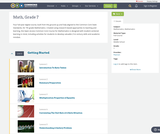Conditions of Use:
Remix and Share
Rating

Four full-year digital course, built from the ground up and fully-aligned to the Common Core State Standards, for 7th grade Mathematics. Created using research-based approaches to teaching and learning, the Open Access Common Core Course for Mathematics is designed with student-centered learning in mind, including activities for students to develop valuable 21st century skills and academic mindset.

Subject:
Mathematics
Material Type:
Full Course
Provider:
Pearson
10/06/2016Conditions of Use:
Remix and Share
Rating

Algebraic Reasoning

Type of Unit: Concept

Prior Knowledge

Students should be able to:

Add, subtract, multiply, and divide rational numbers.
Evaluate expressions for a value of a variable.
Use the distributive property to generate equivalent expressions including combining like terms.
Understand solving an equation or inequality as a process of answering a question: which values from a specified set, if any, make the equation or inequality true?
Write and solve equations of the form x+p=q and px=q for cases in which p, q, and x are non-negative rational numbers.
Understand and graph solutions to inequalities x<c or x>c.
Use equations, tables, and graphs to represent the relationship between two variables.
Relate fractions, decimals, and percents.
Solve percent problems included those involving percent of increase or percent of decrease.

Lesson Flow

This unit covers all of the Common Core State Standards for Expressions and Equations in Grade 7. Students extend what they learned in Grade 6 about evaluating expressions and using properties to write equivalent expressions. They write, evaluate, and simplify expressions that now contain both positive and negative rational numbers. They write algebraic expressions for problem situations and discuss how different equivalent expressions can be used to represent different ways of solving the same problem. They make connections between various forms of rational numbers. Students apply what they learned in Grade 6 about solving equations such as x+2=6 or 3x=12 to solving equations such as 3x+6=12 and 3(x−2)=12. Students solve these equations using formal algebraic methods. The numbers in these equations can now be rational numbers. They use estimation and mental math to estimate solutions. They learn how solving linear inequalities differs from solving linear equations and then they solve and graph linear inequalities such as −3x+4<12. Students use inequalities to solve real-world problems, solving the problem first by arithmetic and then by writing and solving an inequality. They see that the solution of the algebraic inequality may differ from the solution to the problem.

Subject:
Mathematics
Algebra
Material Type:
Unit of Study
Provider:
PearsonConditions of Use:
Remix and Share
Rating

Students explore the effects of wind on a plane's time and distance and represent these situations using algebraic expressions and equations. They use terms with positive, negative, and zero coefficients.Key ConceptsIn this lesson, students show what they remember from Grade 6 about writing expressions and solving one-step equations. They use what they learned earlier in Grade 7 about adding and subtracting integers. They extend these concepts to write and interpret an expression with a negative coefficient.Goals and Learning ObjectivesReview addition and subtraction of integers.Review the relationship between distance, time, and speed.Write an algebraic expression for distance in terms of time, t.Write a term with a negative coefficient.Review solving a one-step equation using the multiplication property of equality.

Subject:
Algebra
Material Type:
Lesson Plan
Provider:
Pearson
09/21/2015Conditions of Use:
Remix and Share
Rating

Working With Rational Numbers

Type of Unit: Concept

Prior Knowledge

Students should be able to:

Compare and order positive and negative numbers and place them on a number line.
Understand the concepts of opposites absolute value.

Lesson Flow

The unit begins with students using a balloon model to informally explore adding and subtracting integers. With the model, adding or removing heat represents adding or subtracting positive integers, and adding or removing weight represents adding or subtracting negative integers.

Students then move from the balloon model to a number line model for adding and subtracting integers, eventually extending the addition and subtraction rules from integers to all rational numbers. Number lines and multiplication patterns are used to find products of rational numbers. The relationship between multiplication and division is used to understand how to divide rational numbers. Properties of addition are briefly reviewed, then used to prove rules for addition, subtraction, multiplication, and division.

This unit includes problems with real-world contexts, formative assessment lessons, and Gallery problems.

Subject:
Mathematics
Algebra
Material Type:
Unit of Study
Provider:
PearsonConditions of Use:
Remix and Share
Rating

Students use the Hot Air Balloon simulation to model integer subtraction. They then move to modeling subtraction on a number line. They use patterns in their work and their answers to write a rule for subtracting integers.Key ConceptsThis lesson introduces the number line model for subtracting integers. To subtract on a number line, start at 0. Move to the location of the first number (the minuend). Then, move in the negative direction (down or left) to subtract a positive integer or in the positive direction (up or right) to subtract a negative integer. In other words, to subtract a number, move in the opposite direction than you would if you were adding it.The Hot Air Balloon simulation can help students see why subtracting a number is the same as adding the opposite:Subtracting a positive number means removing heat from air, which causes the balloon to go down, in the negative direction.Subtracting a negative number means removing weight, which causes the balloon to go up, in the positive direction.The rule for integer subtraction (which extends to addition of rational numbers) is easiest to state in terms of addition: to subtract a number, add its opposite. For example, 5 – 2 = 5 + (–2) = 3 and 5 – (–2) = 5 + 2 = 7.Goals and Learning ObjectivesModel integer subtraction on a number line.Write a rule for subtracting integers.

Subject:
Numbers and Operations
Material Type:
Lesson Plan
Provider:
Pearson
09/21/2015Conditions of Use:
Remix and Share
Rating

Lesson OverviewStudents learn the definition of rational number, and they write rational numbers as ratios of integers and as repeating or terminating decimals.Key ConceptsStudents have been working with rational numbers throughout this unit, but the term rational number is formally defined in this lesson. A rational number is a number that can be written in the form pq, where p and q are integers. All the integers, fractions, decimals, and percents students have worked with so far in their math classes are rational numbers. Following are some rational numbers written as ratios of integers:36=361−1.2=−12105%=5100 −12=−12Any rational number can also be written as a decimal that terminates or that repeats forever in a regular pattern. For example, 35 = 0.6 and 711 = 0.63636363… Repeating decimals are often written with a bar over the digits that repeat. For example, 0.63636363… can be written as 0.63¯.There are numbers that are irrational. These numbers include π and the square root of any whole number that is not a perfect square, such as 2. The decimal form of an irrational number does not terminate, and the digits do not follow a repeating pattern. Students will study irrational numbers in Grade 8.Goals and Learning ObjectivesUnderstand the definition of rational number.Write rational numbers as ratios of integers.Write rational numbers as terminating or repeating decimals.SWD: Students with disabilities may have difficulty working with decimals and fractions, especially moving between the two. If students demonstrate difficulty to the point of frustration, provide direct instruction on the basics for finding equivalent fractions and decimals.ELL: Target and model key language and vocabulary. Specifically, focus on the term rational, as well as terms such as terminate. As you’re discussing the key points, write the words on the board or on large sheets of paper and explain/demonstrate what the words mean. Since these are important points that students will be using throughout the module, write them on large poster board so that students can use them as a reference. Have students record new terms, definitions, and examples in their Notebook.

Subject:
Numbers and Operations
Material Type:
Lesson Plan
Provider:
Pearson
09/21/2015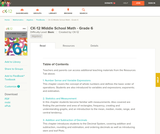Rating

Provides teaching tips, information on common errors, differentiated instruction, enrichment, and problem solving for teachers to use with the CK-12 Middle School Math - Grade 6, Student Edition.

Subject:
Functions
Geometry
Material Type:
Textbook
Provider:
CK-12 Foundation
Provider Set:
CK-12 FlexBook